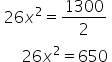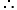Maths-
General
Easy

Question

# The dimensions of a cuboid are in the ratio of 4:3:2 and its total surface area is 1300 cm2 . Find its length, breadth, and height respectively?

## 10 cm , 15 cm, 20 cm15 cm , 10 cm ,20 cm 15 cm , 20 cm , 10 cm20 cm,15cm , 10 cmHint:

## The correct answer is: 20 cm,15cm , 10 cm

### Given: Ratio of dimensions of a cuboid is 4:3:2 & The Total surface area of cuboid is 1300 cm².We have to find Length, breadth & height of cuboid. Let length, breadth and height of cuboid be 4x, 3x and 2x respectively.⠀⠀ Now, As we know that, total surface area of cuboid is given by, Total Surface Area(TSA) of cuboid = 2[ lb + bh + hl ]where, l, b & h are length, breadth and height of cuboid respectively. We have , length = 4x Breadth, b = 3xHeight, h = 2xTSA = 1300 cm2 Putting the values in the formulaDividing both sides of equation by 2Dividing both sides of equation by 26Therefore,The dimensions of cuboid are,Length = 4x = 4(5)= 20Breadth= 3x = 3(5) =15Height = 2x=2(5)= 10Thus, The length, breadth & height of cuboid are 20 cm, 15 cm & 10 cm respectively.Therefore the correct option is d) 20 cm,15cm , 10 cm#### With Turito Foundation.#### Get an Expert Advice From Turito.